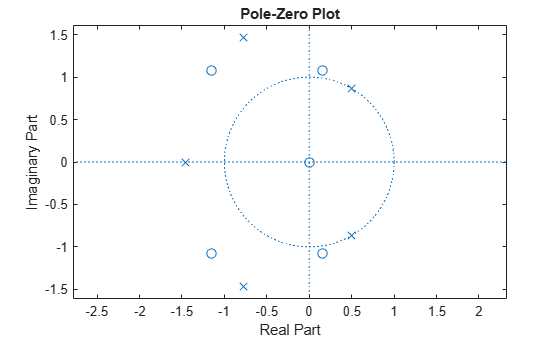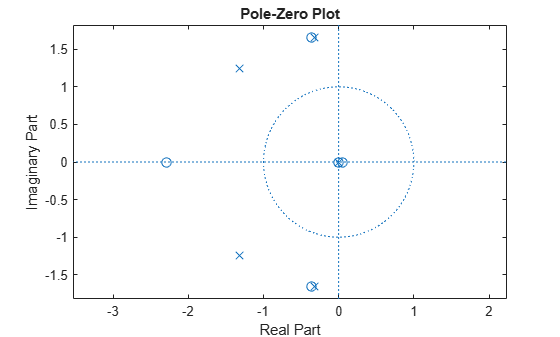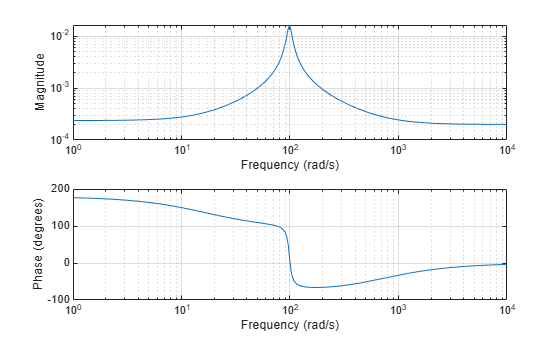invfreqs

Identify continuous-time filter parameters from frequency response data

Description

example

[b,a] = invfreqs(h,w,n,m) returns the real numerator and denominator coefficient vectors b and a of the transfer function h.

[b,a] = invfreqs(h,w,n,m,wt) weights the fit-errors versus frequency using wt.

example

[b,a] = invfreqs(___,iter) provides an algorithm that guarantees stability of the resulting linear system by searching for the best fit using a numerical, iterative scheme. This syntax can include any combination of input arguments from the previous syntaxes.

[b,a] = invfreqs(___,tol) uses tol to decide convergence of the iterative algorithm.

[b,a] = invfreqs(___,'trace') displays a textual progress report of the iteration.

[b,a] = invfreqs(h,w,'complex',n,m,___) creates a complex filter. In this case no symmetry is enforced, and the frequency is specified in radians between –π and π.

Examples

collapse all

Convert a simple transfer function to frequency-response data and then back to the original filter coefficients.

a = [1 2 3 2 1 4];
b = [1 2 3 2 3];

[h,w] = freqs(b,a,64);
[bb,aa] = invfreqs(h,w,4,5)
bb = 1×5

1.0000    2.0000    3.0000    2.0000    3.0000

aa = 1×6

1.0000    2.0000    3.0000    2.0000    1.0000    4.0000

bb and aa are equivalent to b and a, respectively. However, the system is unstable because aa has poles with positive real part. View the poles of bb and aa.

zplane(bb,aa)Use the iterative algorithm of invfreqs to find a stable approximation to the system.

[bbb,aaa] = invfreqs(h,w,4,5,[],30)
bbb = 1×5

0.6816    2.1015    2.6694    0.9113   -0.1218

aaa = 1×6

1.0000    3.4676    7.4060    6.2102    2.5413    0.0001

Verify that the system is stable by plotting the new poles.

zplane(bbb,aaa)Generate two vectors, mag and phase, that simulate magnitude and phase data gathered in a laboratory. Also generate a vector, w, of frequencies.

rng('default')

fs = 1000;
t = 0:1/fs:2;
mag = periodogram(sin(2*pi*100*t)+randn(size(t))/10,[],[],fs);
phase = randn(size(mag))/10;
w = linspace(0,fs/2,length(mag))';

Use invfreqs to convert the data into a continuous-time transfer function. Plot the result.

[b,a] = invfreqs(mag.*exp(1j*phase),w,2,2,[],4);

freqs(b,a)Input Arguments

collapse all

Frequency response, specified as a vector.

Angular frequencies at which h is computed, specified as a vector.

Desired order of the numerator and denominator polynomials, specified as positive integer scalars.

Data Types: single | double

Weighting factors, specified as a vector. wt is a vector of weighting factors that is the same length as w.

Data Types: single | double

Number of iterations in the search algorithm, specified as a positive real scalar. The iter parameter tells invfreqs to end the iteration when the algorithm has converged to a solution, or after iter iterations, whichever occurs first.

Tolerance, specified as a scalar. invfreqs defines convergence as occurring when the norm of the (modified) gradient vector is less than tol.

To obtain a weight vector of all ones, use

invfreqs(h,w,n,m,[],iter,tol)

Output Arguments

collapse all

Transfer function coefficients, returned as vectors. Express the transfer function in terms of b and a as

$H\left(s\right)=\frac{B\left(s\right)}{A\left(s\right)}=\frac{b\left(1\right){s}^{n}+b\left(2\right){s}^{n-1}+\cdots +b\left(n+1\right)}{a\left(1\right){s}^{m}+a\left(2\right){s}^{m-1}+\cdots +a\left(m+1\right)}$

Example: b = [1 3 3 1]/6 and a = [3 0 1 0]/3 specify a third-order Butterworth filter with normalized 3 dB frequency 0.5π rad/sample.

Data Types: double | single
Complex Number Support: Yes

Tips

When building higher order models using high frequencies, it is important to scale the frequencies, dividing by a factor such as half the highest frequency present in w, so as to obtain well-conditioned values of a and b. This corresponds to a rescaling of time.

Algorithms

By default, invfreqs uses an equation error method to identify the best model from the data. This finds b and a in

$\underset{b,a}{\mathrm{min}}\sum _{k=1}^{n}wt\left(k\right){|h\left(k\right)A\left(w\left(k\right)\right)-B\left(w\left(k\right)\right)|}^{2}$

by creating a system of linear equations and solving them with the MATLAB® \ operator. Here A(w(k)) and B(w(k)) are the Fourier transforms of the polynomials a and b, respectively, at the frequency w(k), and n is the number of frequency points (the length of h and w). This algorithm is based on Levi . Several variants have been suggested in the literature, where the weighting function wt gives less attention to high frequencies.

The superior (“output-error”) algorithm uses the damped Gauss-Newton method for iterative search , with the output of the first algorithm as the initial estimate. This solves the direct problem of minimizing the weighted sum of the squared error between the actual and the desired frequency response points.

$\underset{b,a}{\mathrm{min}}\sum _{k=1}^{n}wt\left(k\right){|h\left(k\right)-\frac{B\left(w\left(k\right)\right)}{A\left(w\left(k\right)\right)}|}^{2}$

 Levi, E. C. “Complex-Curve Fitting.” IRE Trans. on Automatic Control. Vol. AC-4, 1959, pp. 37–44.

 Dennis, J. E., Jr., and R. B. Schnabel. Numerical Methods for Unconstrained Optimization and Nonlinear Equations. Englewood Cliffs, NJ: Prentice-Hall, 1983.